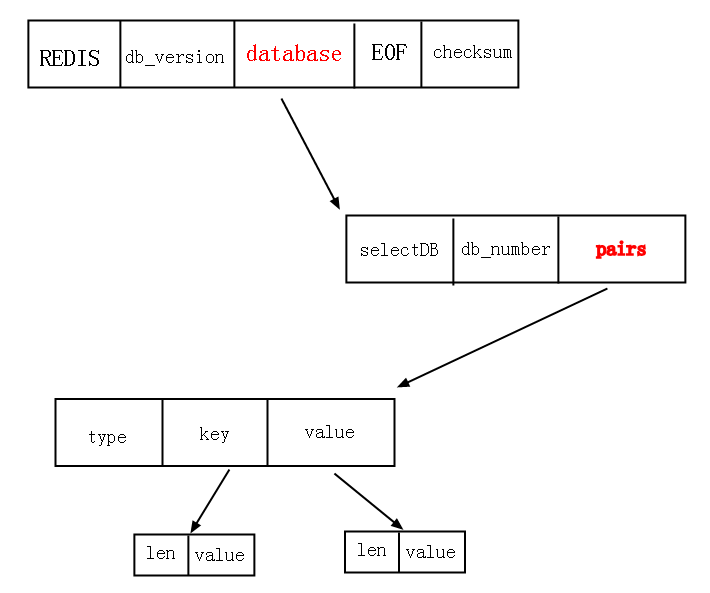## murmur hash，一个更快的hash算法

MurmurHash算法，自称超级快的hash算法，是FNV的4-5倍。官方数据如下：

OneAtATime – 354.163715 mb/sec
FNV – 443.668038 mb/sec
SuperFastHash – 985.335173 mb/sec
lookup3 – 988.080652 mb/sec
MurmurHash 1.0 – 1363.293480 mb/sec
MurmurHash 2.0 – 2056.885653 mb/sec

```    //-----------------------------------------------------------------------------
// MurmurHash2, 64-bit versions, by Austin Appleby

// The same caveats as 32-bit MurmurHash2 apply here - beware of alignment
// and endian-ness issues if used across multiple platforms.

typedef unsigned long int uint64_t;

// 64-bit hash for 64-bit platforms
uint64_t MurmurHash64A ( const void * key, int len, unsigned int seed )
{
const uint64_t m = 0xc6a4a7935bd1e995;
const int r = 47;

uint64_t h = seed ^ (len * m);

const uint64_t * data = (const uint64_t *)key;
const uint64_t * end = data + (len/8);

while(data != end)
{
uint64_t k = *data++;

k *= m;
k ^= k >> r;
k *= m;

h ^= k;
h *= m;
}

const unsigned char * data2 = (const unsigned char*)data;

switch(len & 7)
{
case 7: h ^= uint64_t(data2) << 48;
case 6: h ^= uint64_t(data2) << 40;
case 5: h ^= uint64_t(data2) << 32;
case 4: h ^= uint64_t(data2) << 24;
case 3: h ^= uint64_t(data2) << 16;
case 2: h ^= uint64_t(data2) << 8;
case 1: h ^= uint64_t(data2);
h *= m;
};

h ^= h >> r;
h *= m;
h ^= h >> r;

return h;
}

// 64-bit hash for 32-bit platforms
uint64_t MurmurHash64B ( const void * key, int len, unsigned int seed )
{
const unsigned int m = 0x5bd1e995;
const int r = 24;

unsigned int h1 = seed ^ len;
unsigned int h2 = 0;

const unsigned int * data = (const unsigned int *)key;

while(len >= 8)
{
unsigned int k1 = *data++;
k1 *= m; k1 ^= k1 >> r; k1 *= m;
h1 *= m; h1 ^= k1;
len -= 4;

unsigned int k2 = *data++;
k2 *= m; k2 ^= k2 >> r; k2 *= m;
h2 *= m; h2 ^= k2;
len -= 4;
}

if(len >= 4)
{
unsigned int k1 = *data++;
k1 *= m; k1 ^= k1 >> r; k1 *= m;
h1 *= m; h1 ^= k1;
len -= 4;
}

switch(len)
{
case 3: h2 ^= ((unsigned char*)data) << 16;
case 2: h2 ^= ((unsigned char*)data) << 8;
case 1: h2 ^= ((unsigned char*)data);
h2 *= m;
};

h1 ^= h2 >> 18; h1 *= m;
h2 ^= h1 >> 22; h2 *= m;
h1 ^= h2 >> 17; h1 *= m;
h2 ^= h1 >> 19; h2 *= m;

uint64_t h = h1;

h = (h << 32) | h2;

return h;
}```

## 前言

```php hello.php
```

## 问题描述

```array(3) {
=>
string(5) "HY000"
=>
int(2014)
=>
string(269) "Cannot execute queries while other unbuffered queries are active.  Consider using PDOStatement::fetchAll().  Alternatively, if your code is only ever going to run against mysql, you may enable query buffering by setting the PDO::MYSQL_ATTR_USE_BUFFERED_QUERY attribute."
}
```

## 一：RDB结构剖析## c语言中的define用法

### define基本用法，简单定义

`#define PI 3.141592654`

```#define PI 3.141592654
#include "stdio.h"
int main(){
int r = 3;
float s;
s = PI*r*r;
printf("%f",s);
}```

## varnish安装：varnish如何安装

ubuntu中varnish的安装

varnish在Ubuntu package 仓库版本可能比较低，,我们一般建议使用varnish-cache.org提供的包。请注意，我们只为Ubuntu的LTS版本（ Long Term Support，长时间支持版本，一般三年）提供安装包,其他中间版本并不提供。但这些版本也许会在较新的ubuntu版本中工作。varnish支持的架构是amd64。

``` apt-get install apt-transport-https
curl https://repo.varnish-cache.org/GPG-key.txt | apt-key add -
echo "deb https://repo.varnish-cache.org/ubuntu/ trusty varnish-4.1" >> /etc/apt/sources.list.d/varnish-cache.list
apt-get update
apt-get install varnish```

## varnish的基本工作原理

Varnish是一个HTPP反向代理缓存，它接受来自客户端的请求并试图从缓存中取出相应的数据来应答，如果缓存中并没有相应的数据，它将会把请求指向后端机器，获取并且储存响应的数据，之后再交付给用户。

## XMLHttpRequest文档

`XMLHttpRequest` 是一个 JavaScript 对象,它最初由微软设计,随后被 Mozilla,Apple, 和 Google采纳. 如今,该对象已经被 W3C组织标准化. 通过它,你可以很容易的取回一个URL上的资源数据. 尽管名字里有XML, 但`XMLHttpRequest` 可以取回所有类型的数据资源,并不局限于XML. 而且除了HTTP ,它还支持`file``ftp` 协议.

``var req = new XMLHttpRequest();``

## 方法概述

非标准方法 `void abort();` `DOMString getAllResponseHeaders();` `DOMString? getResponseHeader(DOMString header);` `void open(DOMString method, DOMString url, optional boolean async, optional DOMString? user, optional DOMString? password);` `void overrideMimeType(DOMString mime);` `void send();` `void send(ArrayBuffer data);` `void send(Blob data);` `void send(Document data);` `void send(DOMString? data);` `void send(FormData data);` `void setRequestHeader(DOMString header, DOMString value);` `[noscript] void init(in nsIPrincipal principal, in nsIScriptContext scriptContext, in nsPIDOMWindow ownerWindow);` `[noscript] void openRequest(in AUTF8String method, in AUTF8String url, in boolean async, in AString user, in AString password); ` `void sendAsBinary(in DOMString body);`

## ajax的核心，好好认识一下XMLHttpRequest

### 从new一个对象开始

`var xmlhttp = new XMLHttpRequest();`（更多…）